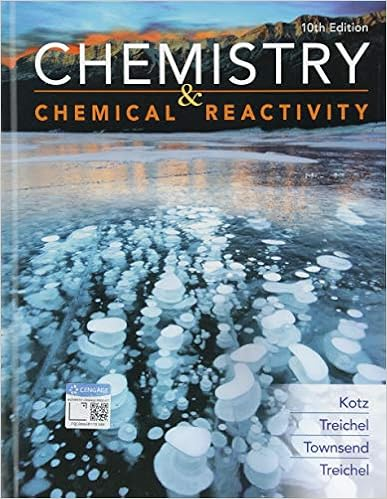# From equation 385 the heat loss is hence for copper

• Test Prep
• 16
• 100% (2) 2 out of 2 people found this document helpful

This preview shows page 7 - 9 out of 16 pages.

##### We have textbook solutions for you!
The document you are viewing contains questions related to this textbook.The document you are viewing contains questions related to this textbook.
Chapter 5 / Exercise 104
Chemistry & Chemical Reactivity
Kotz/TreichelExpert Verified
From Equation 3.85, the heat loss is Hence for copper, Similarly, for the aluminum alloy and stainless steel, respectively, the heat rates are q f 5.6 W and 1.6 W. 2. Since there is no heat loss from the tip of an infinitely long rod, an estimate of the validity of this approximation may be made by comparing Equations 3.81 and 3.85. To a satisfactory approximation, the expressions provide equivalent results if tanh mL 0.99 or mL 2.65. Hence a rod may be assumed to be infinitely long if For copper, Results for the aluminum alloy and stainless steel are L 0.13 m and L 0.04 m, respectively. L 2.65 398 W/m K ( /4)(0.005 m) 2 100 W/m 2 K (0.005 m) 1/2 0.19 m L L 2.65 m 2.65 kA c hP 1/2 8.3 W 398 W/m K 4 (0.005 m) 2 1/2 (100 25) C q f 100 W/m 2 K 0.005 m q f hPkA c b T ( ° C) 100 300 250 200 150 100 50 0 20 40 80 60 T Cu 2024 Al 316 SS x (mm) 3.6 Heat Transfer from Extended Surfaces 163
##### We have textbook solutions for you!
The document you are viewing contains questions related to this textbook.The document you are viewing contains questions related to this textbook.
Chapter 5 / Exercise 104
Chemistry & Chemical Reactivity
Kotz/TreichelExpert Verified
Comments: 1. The foregoing results suggest that the fin heat transfer rate may accurately be predicted from the infinite fin approximation if mL 2.65. However, if the infinite fin approxi- mation is to accurately predict the temperature distribution T ( x ), a larger value of mL would be required. This value may be inferred from Equation 3.84 and the requirement that the tip temperature be very close to the ﬂuid temperature. Hence, if we require that ( L )/ b exp( mL ) 0.01, it follows that mL 4.6, in which case L 0.33, 0.23, and 0.07 m for the copper, aluminum alloy, and stainless steel, respectively. These results are consistent with the distributions plotted in part 1. 2. This example is solved in the Advanced section of IHT . 3.6.3 Fin Performance Recall that fins are used to increase the heat transfer from a surface by increasing the effec- tive surface area. However, the fin itself represents a conduction resistance to heat transfer from the original surface. For this reason, there is no assurance that the heat transfer rate will be increased through the use of fins. An assessment of this matter may be made by evaluating the fi n effectiveness f . It is defined as the ratio of the fi n heat transfer rate to the heat transfer rate that would exist without the fi n. Therefore (3.86) where A c , b is the fin cross-sectional area at the base. In any rational design the value of f should be as large as possible, and in general, the use of fins may rarely be justified unless f 2. Subject to any one of the four tip conditions that have been considered, the effectiveness for a fin of uniform cross section may be obtained by dividing the appropriate expression for q f in Table 3.4 by hA c , b b . Although the installation of fins will alter the surface convection coefficient, this effect is commonly neglected. Hence, assuming the convection coefficient of the finned surface to be equivalent to that of the unfinned base, it follows that, for the infi- nite fin approximation (Case D), the result is (3.87) Several important trends may be inferred from this result. Obviously, fin effectiveness is enhanced by the choice of a material of high thermal conductivity. Aluminum alloys and copper come to mind. However, although copper is superior from the standpoint of thermal
•••